# 6 Computation of higher-order deformations

With the first-order deformation at hand, in the sequel we determine the higher-order deformations of the solution to the master equation, governed by equations (59)-(61), etc., which comply with our working hypotheses.

In the first step we approach the second-order deformation,, as (nontrivial) solution to equation (59). If we denote by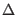the nonintegrated density of the antibracketand bythe nonintegrated density associated with,(149)

then equation (59) takes the local form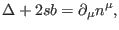(150)

with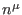a local current. By direct computation it follows thatdecomposes as(151)

where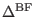involves only BRST generators from the BF sector and each term fromdepends simultaneously on the BRST generators of both sectors (BF and mixed symmetry), such thatcouples the two theories. Consequently, decomposition (125) induces a similar one at the level of the second-order deformation(152)

and equation (124) becomes equivalent to two equations, one for the BF sector and the other for the interacting sector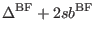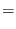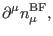(153)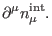(154)

Equation (127) has been completely solved in , where it was shown that it possesses only the trivial solution(155)

and, in addition, the seven functions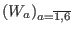and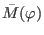that parameterizeare subject to the following equations: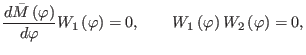(156)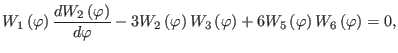(157)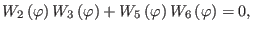(158)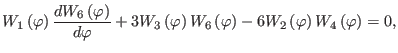(159)(160)(161)

Now, we investigate the latter equation, (128). By direct computationcan be brought to the form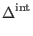(162)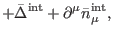(163)

whereis a local current and(164)

In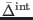we used the notations(165)(166)

and the polynomialsare listed in Appendix E (see formulas (264)-(275)). It can be shown that (137) cannot be written as a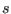-exact modulo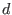element from local functions and therefore it must vanish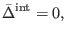(167)

which further restricts the functions and constants that parameterize the first-order deformation to obey the supplementary equations(168)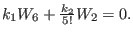(169)

As a consequence, the consistency of the first-order deformation at order two in the coupling constant (the existence of local solutions to equation ( 59)) on the one hand restricts the functions and constants that parameterize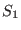to fulfill equations (130)-(135) and ( 141)-(142) and, on the other hand, enables us (via formulas ( 123), (126), (128), (129), (136 ), and (140)) to infer the second-order deformation as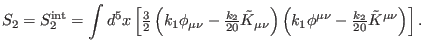(170)

In the second step we solve the equation that governs the third-order deformation, namely, (60). If we make the notations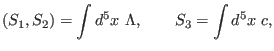(171)

then equation (60) takes the local form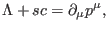(172)

with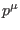a local current. By direct computation we obtain(173)

whereis a local current and the functionsappearing in the right-hand side of (146) are listed in Appendix E (see formulas (276)-(284)). Taking into account the result that the functions and constants that parameterize both the first- and second-order deformations satisfy equations (130)-( 135) and (141)-(142) and comparing (146) with equation (145), it results that the third-order deformation can be chosen to be completely trivial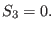(174)

Related to the equation that governs the fourth-order deformation, namely, ( 61), we have that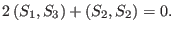(175)

From (148) and (61) we find that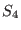is completely trivial(176)

Along a similar line, it can be shown that all the remaining higher-order deformations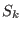() can be taken to vanish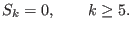(177)

The main conclusion of this section is that the deformed solution to the master equation for the model under study, which is consistent to all orders in the coupling constant, can be taken as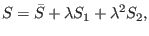(178)

where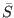reads as in (53),is given in (121) withof the form (122), andis expressed by (143). It represents the most general solution that complies with all our working hypotheses (see the discussion from the beginning of section 4). We cannot stress enough that the (seven) functions and (two) constants that parameterize the fully deformed solution to the master equation are no longer independent. They must obey equations ( 130)-(135) and (141)-(142).

Ashkbiz Danehkar
2018-03-26